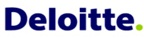# Placement Papers - DeloitteDeloitte Interview Pattern - TSEC, BANDRA(W) August 13, 2016
Posted by :
Sameedha Salvi
(464)
There were 3 sections:

VERBAL -> 25 questions - 25 mins.
QUANTS -> 25 questions - 35mins.
LOGICAL -> 24 questions - 35 mins.

VERBAL QUESTIONS:
2 Reading Comprehension, Synonyms, Antonyms, Fill in the blanks, Ordering of sentences.

QUANTS QUESTIONS:

1) log(10x) = ?

2) Log to the base 11 (1000+x) = 3.

3) log 64 to the base 16 - log 16 to the base 64.

4) Length of the rectangle field is 4 times the breadth. The area of rectangular field is 2500 Yards. Find the length of the field.

5) Find the probability of getting at least one tail if two coins are tossed simultaneously.

6) There are 4 raspberries and 8 strawberries. Find the probability if two fruits are chosen and both are raspberries.

7) If b is the simple interest on a and c is the simple interest on b at same period and same rate of interest. Find the relation between a, b and c.

8) There are 2 posts and 12 equally qualified candidates. Find the number of ways in which they can be selected?

9) In how many ways can the letters of the word SYSTEM be arranged so that E is always at the third position?

10) What power of 5 is contained in 200! ?

11) The price of Onions soared up by 15% and so Shiny decreased the consumption of onions by 10%. Find the percentage decrease in her expenses.

12) In how many ways can the word ARRANGE be arranged so that the two R\'s are never together?

13) x= 22^22 , x=2^222 , x= 222^2 Which value of x is the greatest?

14) The reciprocal of HCF and LCM of two numbers is 1/12 and 1/312 respectively. If one of the number is 24, find the other.

15) If the square root of HCF of two numbers is 2 and LCM is 2516, find the product of the 2 numbers.

LOGICAL QUESTIONS:

1) If a man goes 20 kms to the South and then 15 kms to the east. At what distance he is from the starting position?

2) If Northwest is East, Northeast is South, then what does East represent?

3) Find the next number in the series 2, 3, 6, 18, 108, .....

4)Statement : product of two numbers u and v is 42. Find the two numbers.
i) u is less than v
ii) u is even
a) i is only sufficient b) ii is only sufficient c) both i and ii are necessary d) both i and ii together are not sufficient.

5) a) P, Q, R, S, T are 5 friends.
b) Each one plays only one game out of cricket, hockey, tennis, chess and badminton
c) Each one has a different colour car out of white, green, black, blue and red
d) S plays cricket and has a red colour car.
e) Q does not like either chess or badminton and does not have either blue, black or red car.
f) T plays badminton and has a black car.
g) P plays tennis and has a green car.

1] Who plays hockey?
2] Who has a white car?

6) How is Mr. Sharma related to Santosh?
i. Santosh\'s mom has 2 daughters.
ii. Santosh\'s sister is married to Sharma\'s son.
A) only i is sufficient B) only ii is sufficient C) both are necessary. D) Neither is necessary.

PS: practice verbal and logical from Indiabix and quants from both Indiabix and RS Agarwal.

Thank you!

Hope this helps you guys by giving a fair idea of the paper pattern!